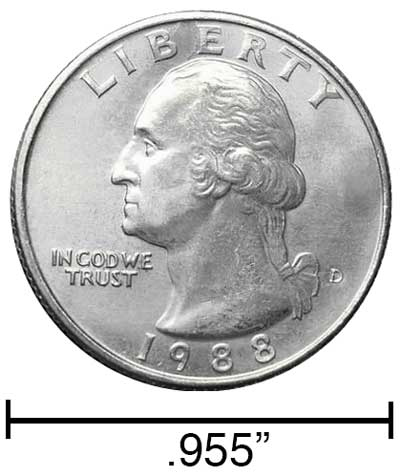# Inches to Meters Conversion

Enter the length in inches below to get the value converted to meters.

/
Results in Meters:1 in = 0.0254 m
Do you want to convert meters to inches?

## How to Convert Inches to MetersTo convert an inch measurement to a meter measurement, multiply the length by the conversion ratio.

Since one inch is equal to 0.0254 meters, you can use this simple formula to convert:

meters = inches × 0.0254

The length in meters is equal to the inches multiplied by 0.0254.

For example, here's how to convert 5 inches to meters using the formula above.
5" = (5 × 0.0254) = 0.127 m

Our inch fraction calculator can add inches and meters together, and it also automatically converts the results to US customary, imperial, and SI metric values.

## Inches

An inch is a unit of linear length measure equal to 1/12 of a foot or 1/36 of a yard. Because the international yard is legally defined to be equal to exactly 0.9144 meters, one inch is equal to 2.54 centimeters.

The inch is a US customary and imperial unit of length. Inches can be abbreviated as in; for example, 1 inch can be written as 1 in.

Inches can also be denoted using the symbol, otherwise known as a double-prime. Often a double-quote (") is used instead of a double-prime for convenience. A double-prime is commonly used to express 1 in as 1″.The standard ruler has 12", and is a common measuring tool for measuring inches. They are also often measured using tape measures, which commonly come in lengths from 6' - 35'. Other types of measuring devices include scales, calipers, measuring wheels, micrometers, yardsticks, and even lasers.

## Meters

The meter is defined as the length of the path traveled by light in vacuum during a time interval with a duration of 1/299,792,458 of a second, according to the most recent 2019 definition. One meter is equal to 100 centimeters or 39.37 inches.

The meter, or metre, is the SI base unit for length in the metric system. Meters can be abbreviated as m; for example, 1 meter can be written as 1 m.

We recommend using a ruler or tape measure for measuring length, which can be found at a local retailer or home center. Rulers are available in imperial, metric, or combination with both values, so make sure you get the correct type for your needs.

Need a ruler? Try our free downloadable and printable rulers, which include both imperial and metric measurements.

## Inch to Meter Conversion Table

Inch measurements converted to meters
Inches Meters
1" 0.0254 m
2" 0.0508 m
3" 0.0762 m
4" 0.1016 m
5" 0.127 m
6" 0.1524 m
7" 0.1778 m
8" 0.2032 m
9" 0.2286 m
10" 0.254 m
11" 0.2794 m
12" 0.3048 m
13" 0.3302 m
14" 0.3556 m
15" 0.381 m
16" 0.4064 m
17" 0.4318 m
18" 0.4572 m
19" 0.4826 m
20" 0.508 m
21" 0.5334 m
22" 0.5588 m
23" 0.5842 m
24" 0.6096 m
25" 0.635 m
26" 0.6604 m
27" 0.6858 m
28" 0.7112 m
29" 0.7366 m
30" 0.762 m
31" 0.7874 m
32" 0.8128 m
33" 0.8382 m
34" 0.8636 m
35" 0.889 m
36" 0.9144 m
37" 0.9398 m
38" 0.9652 m
39" 0.9906 m
40" 1.016 m

## References

1. National Institute of Standards and Technology, Checking the Net Contents of Packaged Goods, Handbook 133 - 2019 Edition, https://nvlpubs.nist.gov/nistpubs/hb/2019/NIST.HB.133-2019.pdf
2. International Bureau of Weights and Measures, The International System of Units, 9th Edition, 2019, https://www.bipm.org/documents/20126/41483022/SI-Brochure-9-EN.pdf